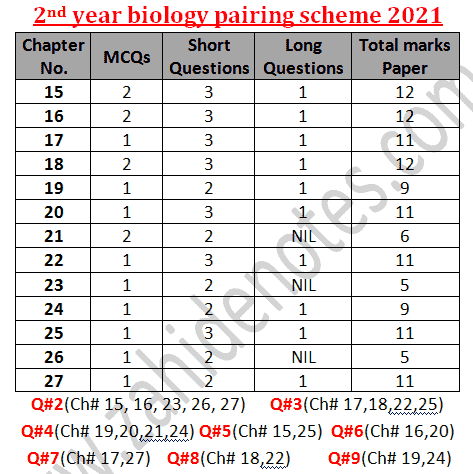The biology scheme for 2021 for 2nd year is a scheme that helps the students understand what is important in the book. It allows you to define your priority set up if you are going to prepare for your final exams in a very short time. The biology of 2nd year has 13 chapters and the weightage of marks is according to this scheme for 2021 board exams.

# 2nd year biology paper scheme 2021

Now let me tell you all things in details. Here are the ingredients of a complete scheme in details. Meanwhile you may also see the following posts:
In other words, the picture of the pairing will allow you to easily download it in mobile. The texts will allow you to copy-paste it or share it as SMS.

## 12th class biology new pairing scheme 2021 Punjab boards

The new paper scheme for 2nd year biology 2021 is given below in image form. Some students prepare for the exams according to the specific scheme. This scheme helps the students to get high marks in shor time. The planned study program can be made with the help of this scheme.## 2nd year biology chapterwise long and short questions

Question No. 1 MCQs = 17 (1 mcqs from chapter 17, 19, 20, 22, 23, 24, 25, 26, and 27 each and 2 mcqs from chapter 15, 16, 18 and 21 each)

Question No. 2 (8/12)
Chapter 15 = 3 short questions
Chapter 16 = 3 short questions
Chapter 23 = 2 short questions
Chapter 26 = 2 short questions
Chapter 27 = 2 short questions

Question No. 3 (8/12)
Chapter 17 = 3 short questions
Chapter 18 = 3 short questions
Chapter 22 = 3 short questions
Chapter 25 = 3 short questions

Question No. 4 (6/9)
Chapter 19 = 2 short questions
Chapter 20 = 3 short questions
Chapter 21 = 2 short questions
Chapter 24 = 2 short questions

Long questions (Attempt ANY THREE questions from FIVE Questions)

Questions No. 5
Chapter 15 = part A
Chapter 25 = Part B

Questions No. 6
Chapter 16 = part A
Chapter 20 = Part B

Questions No. 7
Chapter 17 = part A
Chapter 27 = Part B

Questions No. 8
Chapter 18 = part A
Chapter 22 = Part B

Questions No. 9
Chapter 19 = part A
Chapter 24 = Part B

Relevant posts:
2nd year biology pairing scheme 2021Reviewed by Saif Ullah ZahidonJanuary 26, 2021 Rating: 5

1.Sir is it new

2.vibes good thanks.

3.Please tell the pairing scheme of fedral board also

4.Sir ab 3 paper hon gy to please bta dain ab scheme ho gi ya nahi???

1.5.Any reason... Why are some chapters underlined?